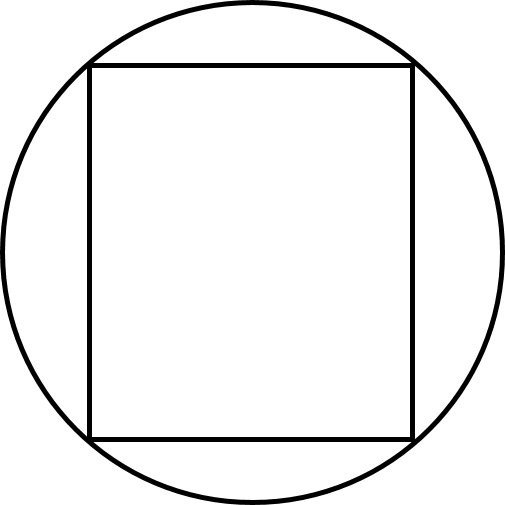# Inscribed Rectangle

Geometry Level 3

A rectangle is inscribed within a circle of radius $7\text{ cm}$, and this rectangle has a perimeter $32\text{ cm}$. What is the area of this rectangle?×